Quantity: 0

Total: 0,00

0

# Szilassi polyhedron

### Szilassi polyhedron

This special concave polyhedron was named after a Hungarian mathematician.

Mathematics

Keywords

Szilassi polyhedron, polyhedrons, face, vertex, edge, neighbouring faces, solids, geometry, mathematics

Related items

### Scenes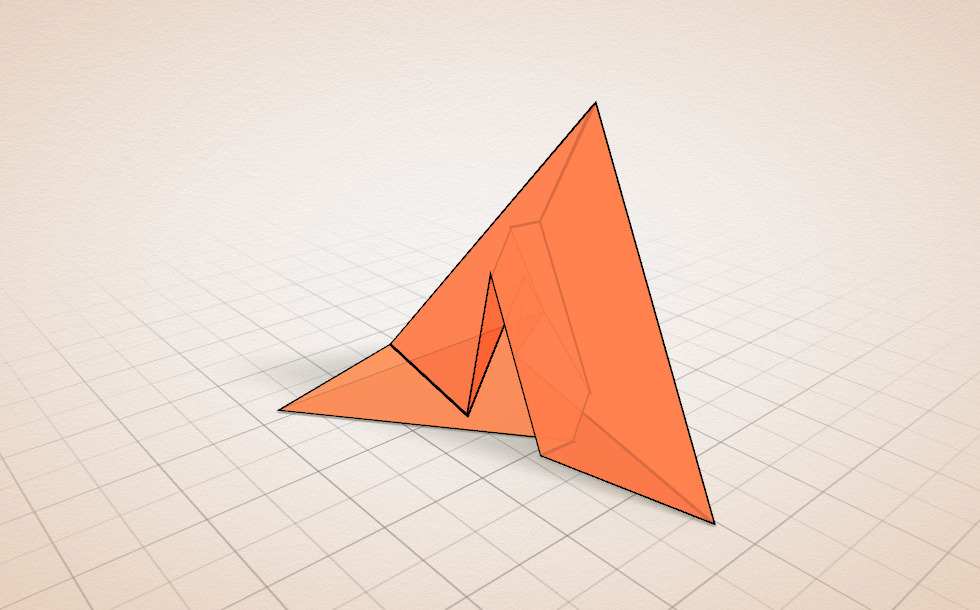### Szilassi solid

This special solid was invented by Hungarian mathematician Lajos Szilassi.

It is a polyhedron, i.e. it only consists of polyhedral faces.

Its distinctive feature is that, - according to current knowledge, - with the exception of the tetrahedron, it is the only polyhedron whose any two faces are adjacent, i.e. they share a common vertex.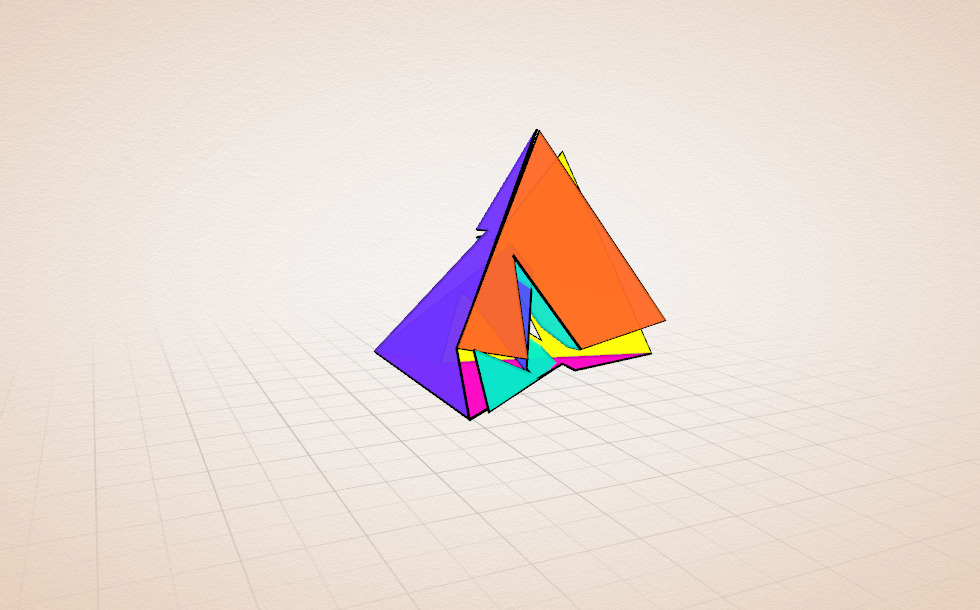### Faces

Number of faces: 7 (hexagonal)
Number of vertices: 14
Number of edges: 21

It is not convex. (A convex polyhedron is a polyhedron whose every point lies in one of the half-spaces determined by the plane of each of its faces.)

It is not simple. It is the dual polyhedron of the Császár polyhedron.

### Related items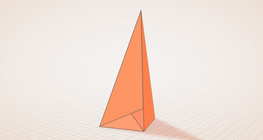#### Császár polyhedron

The Császár polyhedron is a nonconvex polyhedron with 14 triangular faces.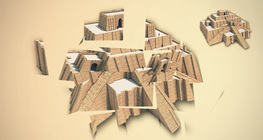#### 3D rotating game

Assemble fragments of broken three-dimensional shapes by rotating the models.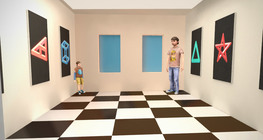#### Ames room

An Ames room is a distorted room that is used to create an optical illusion.#### Conic solids

This animation demonstrates various types of cones and pyramids.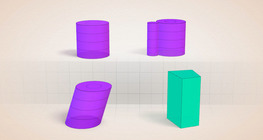#### Cylindrical solids

This animation demonstrates various types of cylindrical solids as well as their lateral surfaces.#### Optical illusion

The information gathered by the eye is misinterpreted by the brain.#### Sphere

A sphere is the set of points which are all within the same distance from a given point in space.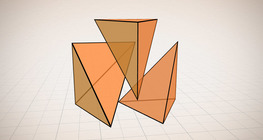#### Volume of a tetrahedron

To calculate the volume of a tetrahedron we start by calculating the volume of a prism.#### Grouping of solids 1

This animation demonstrates various groups of solids through examples.#### Grouping of solids 2

This animation demonstrates various groups of solids through examples.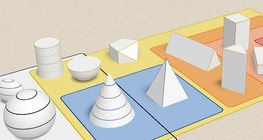#### Grouping of solids 3

This animation demonstrates various groups of solids through examples.#### Grouping of solids 4

This animation demonstrates various groups of solids through examples.#### Platonic solids

This animation demonstrates the five regular three-dimensional (or Platonic) solids, the best known of which is the cube.#### Non-orientable surfaces

The Möbius strip and the Klein bottle are special two-dimensional surfaces with only one side.